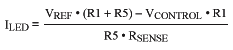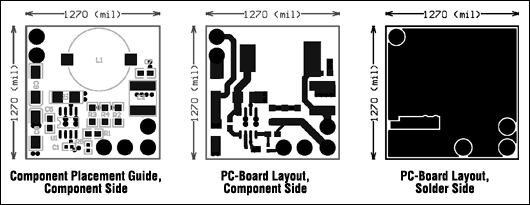# High-Efficiency Current Drive for High-Brightness LEDs

### Abstract

This article describes a simple circuit for regulating the current through high-brightness LEDs. The design includes an off-the-shelf, highly integrated step-down switching regulator (the MAX5035), which allows accurate control of the LED current. The MAX5035 DC/DC converter operates on a fixed frequency of 125kHz with wide 7.5V to 76V input-voltage range especially useful for automotive applications. For brightness control, this converter implements either analog (linear dimming) or low-frequency duty-cycle modulation (PWM dimming).

### Background on High-Brightness LEDs

In recent years, high-brightness (HB) LEDs have gained prominence as the lighting source for a variety of applications. HB LEDs are rugged and reliable semiconductor devices capable of several tens of thousands of cycles—up to 100,000 hours of operation. That performance represents an operating life that is orders of magnitude longer than conventional incandescent and halogen lamps. Thus, HB-LED applications can be found in automotive lighting, public and commercial signage, and architectural lighting.

HB LEDs are PN-junction devices especially processed to produce white, red, green, and blue light when forward biased. (Amber and a few other colors are possible as well.) As PN-junction devices, LEDs exhibit V-I characteristics similar to those of conventional diodes, but with higher voltage drops across their junctions. Little current passes through an LED until the forward voltage reaches VF, which varies from 2.5V for red LEDs to about 4.5V for blue LEDs. When VF is reached, the current increases very rapidly (as in conventional diodes). Consequently, the designer must employ current limiting to prevent possible damage. Current limiting can be implemented with three basic methods, each with advantages and disadvantages (Table 1).

Table 1. Comparison of Current-Limiting Methods

 Current-Limit Method Advantages Disadvantages Power Loss in Series Device¹ Resistor Inexpensive Only one, large component It cannot control current accurately.Current varies to some extent with supply voltage.High power dissipation in the resistor; must be sized accordingly. 2.8W Active Linear Control Linear control-loop circuitry accurately controls the LED current.Allows dimming through current control.Allows dimming through control of amplitude or low-frequency PWM.Allows automatic temperature compensation of LED characteristics. Is more expensive than the simple resistive current limiterFor the same supply voltage, dissipates about the same power as a resistor limiter.May require mechanical heatsinking of the active pass device. 2.8W Switching Regulator Control Control loop regulates LED current precisely.Allows dimming by amplitude control or low-frequency PWM.Allows automatic temperature compensation of LED characteristics.Allows efficient operation over a wide input-voltage range.Does not usually have mechanical heatsinking, which saves cost and complexity.Powers LEDs in applications for which higher input voltage and higher LED currents may cause prohibitive losses with other methods. Has higher cost than the resistor and linear-control methods.Careful design is necessary to allay EMI considerations.Active circuitry may require more area, but the other methods may require more hardware and physical space for cooling. <0.8W

¹Assumes a white LED with VF = 4V, ILED = 350mA, and VIN = 12V.

### Switching Power Supply for HB LEDs

An HB-LED power supply (Figure 1) is based on a fixed-frequency, highly integrated PWM switching converter (MAX5035) rated for output currents to 1A. A similar device (MAX5033) is rated to 500mA. These inductor-based buck-regulator ICs accurately control the current through an LED or series-connected string of LEDs with total voltage up to 12V. The MAX5035 switching frequency is 125kHz, and its input-voltage range extends to 76V. (The higher input voltages require higher ratings for the input capacitor and diode.) Thus, the circuit maintains the LED current constant over a wide range of input voltages. Table 2 summarizes the specifications of this circuit design.Figure 1. Varying the control voltage from 0V to 3.9V causes the MAX5035 LED-current driver to produce an output current from 350mA to 0mA (approximately) between the LED_A and LED_K terminals.

Table 2—Parameters of the Circuit in Figure 1

 Parameter Value Minimum Input Voltage (V) 7.5 (with most single LEDs) Maximum Input Voltage (V) 30 (limited by D1, C8, and C9) Maximum Output Current (mA) 350 (VCONTROL= 0V) Maximum Output Voltage (V) 12 (350mA output, internally limited by MAX5035) Control Voltage Range (VCONTROL) (V) 0 (full current) to 3.9 (fully dimmed)

The control of LED current is achieved by applying voltage to the control terminal of the Figure 1 circuit (Figure 2). Figure 3 shows the efficiency for this control technique.Figure 2. LED current vs. control voltage for the circuit in Figure 1, obtained by connecting an ammeter directly between the LED_A and LED_K terminals.Figure 3. Efficiency vs. LED current for the circuit in Figure 1 driving one, two, and three green 350mA LEDs in series.

The control voltage is summed with the current-sense voltage, developed across the three parallel-connected current-sense resistors, and applied to the IC's feedback terminal (FB). The IC's internal control loop then maintains voltage on the FB pin constant at approximately 1.22V. Thus, a higher control voltage results in lower current, because the sum of the control and current-sense voltages (scaled by R1 and R5) must always equal 1.22V.

The following equation lets you design for output currents and control voltages other than those in the example above:where VREF = 1.22V and RSENSE (the parallel equivalent of R2, R3 and R4) equals 5Ω.

In many cases it is advantageous to dim an LED by pulsing its current at a low frequency (50Hz to 200Hz) and controlling the width of the pulses (Figure 4). Though the LED illuminates with the same brightness during each pulse, the eye perceives a dimming as the pulse narrows. The light spectrum, moreover, remains constant, unlike the case of dimming by amplitude modulation in which the light spectrum shifts as the LED current varies.Figure 4. Data show the control and LED-current waveforms for low-frequency PWM dimming in the circuit in Figure 1. CH1: VCONTROL; Ch3: ILED. The load voltage (three green LEDs in series) is approximately 9.5V. Substituting a smaller output capacitor can reduce the current turn-off tail.

Figure 4 shows the LED current pulsed at 100Hz in response to a squarewave control waveform ranging from 0V to approximately 3.9V. For such low-frequency PWM dimming, the typical efficiency is higher than for the linear-current approach shown in Figure 2.Figure 5. PCB layout for the circuit in Figure 1.

### Conclusion

The IC in Figure 1 (MAX5035 or MAX5033) offers an efficient and cost-effective way to generate a constant-current source for driving high-brightness LEDs. Some of the benefits of that circuit design are summarized below:

• High-Frequency Switching (125kHz) Allows Small Reactive Components (L1 and C2)
• High Efficiency over a Wide Input-Voltage Range
• Output Compliance Voltage (Up to 12V) Accommodates as Many as Three Series-Connected Green HB LEDs
• No Mechanical Heatsinks Required
• Voltage Range Can Be Extended to 76V to Accommodate Automotive HB-LED Applications
• Can Be Used in 24V Signage and Architectural Lighting Applications
• Output Current Can Be Extended to 1A by Changing the Value of Current-Sense Resistors R2, R3, and R4
• High-Integration Power IC Includes Onchip Switching Power MOSFET
• Linear Amplitude Modulation (Linear Dimming) of LED Current Through The Control Input
• Low-Frequency PWM Dimming Through the Control Input
A similar article appeared on CMP's Automotive DesignLine web site.